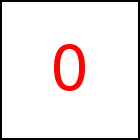# Matrix Methods

taccgl™ includes various matrix operations. Each matrix is represented as a javaScript array. A 3x3 matrix is represented as an array with 9 elements indexed 0..8 and a 4x4 matrix as an array with 16 elements indexed from 0..15. Normally a matrix is stored in row major order, i.e. m contains the first element of the first row and m contains the second element of the first row.

Using the representation it is easily possible to create a matrix using javaScript e.g. m=[1,0,0, 0,1,0, 0,0,1] is the 3x3 identity matrix.

Some matrix functions create a new a javascript object for the result (copying functions) while other matrix functions (in-place funcitions) in-place modify one of the array objects passed to it as parameter. The latter is useful to reduce the number of array objects objects created, but needs careful programming since often one might not expect that these functions change their arguments. When using these latter functions it is neccessary to copy the array object of a matrix, which can be done using the javaScript slice function e.g. m.slice(0).

Vector functions usually start with 'v', matrix functions with 'm'. In-place functions then use an 'i' in their name, copying functions do not. Some functions handle arbitrary sized vectors or matrices, others fixed size. Fixed size functions have 32, 33, or 44 in their name for 4x2, 3x3, or 4z4 matrices.

# m33I Library Method

Short Description: 3x3 Identity Matrix

Signature: taccgl.m33I
Class: taccgl Class

Creates and returns a 3x3 identity matrix.



Class: taccgl Class

Adds the 3x3 matrix b on the matrix a. It allocates a new matrix object for the result and does not modify its arguments.



Short Description: 4x4 Translation Matrix

Class: taccgl Class

Adds the 3x3 matrix b on the matrix a. It modifies the matrix a thereby and returns the modified matrix a.



# m33Mul Library Method

Short Description: 3x3 Matrix Multiplication

Signature: taccgl.m33Mul (a,b)
Class: taccgl Class

Multiplies the 3x3 matrix a with the matrix b. It allocates a new matrix object for the result and does not modify its arguments.



# mi33Mul Library Method

Short Description: 4x4 Translation Matrix

Signature: taccgl.mi33Mul (a,b)
Class: taccgl Class

Multiplies the 3x3 matrix a with the matrix b. It modifies the matrix a thereby and returns the modified matrix a.



# m33T Library Method

Short Description: Transpose 3x3 Matrix

Signature: taccgl.m33T (a)
Class: taccgl Class

Transposes the 3x3 matrix a and returns it. It allocates a new matrix object for the result and does not modify its arguments.



# mi33T Library Method

Short Description: 4x4 Translation Matrix

Signature: taccgl.mi33T (a)
Class: taccgl Class

Transposes the 3x3 matrix a and returns it. Note that this function modifies the matrix object a and does not create a new matrix.



# m33Inverse Library Method

Short Description: 3x3 Matrix Inverse

Signature: taccgl.m33Inverse (a)
Class: taccgl Class

Returns a new matrix contains the inverse matrix of the 3x3 matrix a.



# m33Rotation Library Method

Short Description: 3x3 Rotation Matrix

Signature: taccgl.m33Rotation (alpha,va)
Class: taccgl Class

Returns a rotation matrix around the va axis and the angle alpha. alpha must be given in radians.



# m44I Library Method

Short Description: 4x4 Identity Matrix

Signature: taccgl.m44I
Class: taccgl Class

Creates and returns a 4x4 identity matrix.



Class: taccgl Class

Adds the 4x4 matrix b on the matrix a. It allocates a new matrix object for the result and does not modify its arguments.

Short Description: 4x4 Translation Matrix

Class: taccgl Class

Adds the 4x4 matrix b on the matrix a. It modifies the matrix a thereby and returns the modified matrix a.

# m44Mul Library Method

Short Description: 4x4 Matrix Multiplication

Signature: taccgl.m44Mul (a,b)
Class: taccgl Class

Multiplies the 4x4 matrix a with the matrix b. It allocates a new matrix object for the result and does not modify its arguments.

# mi44Mul Library Method

Short Description: 4x4 Translation Matrix

Signature: taccgl.mi44Mul (a,b)
Class: taccgl Class

Multiplies the 4x4 matrix a with the matrix b. It modifies the matrix a thereby and returns the modified matrix a.

# m44MulV Library Method

Short Description: 4x4 Matrix and Vector Multiplication

Signature: taccgl.m44MulV (a,v)
Class: taccgl Class

Multiplies the 4x4 matrix a with the vector v. v must have exaclty 4 elements. The function returns a new vector of 4 elements.

# m44T Library Method

Short Description: Transpose 4x4 Matrix

Signature: taccgl.m44T (a)
Class: taccgl Class

Transposes the 4x4 matrix a and returns it. It allocates a new matrix object for the result and does not modify its arguments.

# mi44T Library Method

Short Description: 4x4 Translation Matrix

Signature: taccgl.mi44T (a)
Class: taccgl Class

Transposes the 4x4 matrix a and returns it. Note that this function indeed modifies the matrix object a and does not create a new matrix.

# m44FromM33 Library Method

Short Description: Extend 3x3 Matrix to 4x4 Matrix

Signature: taccgl.m44FromM33 (a,x,y,z)
Class: taccgl Class

The parameter a must be a 3x3 Matrix and x,y,z numbers. The 3x3 matrix a is converted into a 4x4 matrix by adding a 4th row containing [0,0,0,1] and a 4th column containing [x,y,z,1].



# m33FromM44 Library Method

Short Description: Take 3x3 Matrix from 4x4 Matrix

Signature: taccgl.m33FromM44 (a,x,y,z)
Class: taccgl Class

The parameter a must be a 4x4 Matrix. It is converted into a 3x3 matrix by removing the last row and the last column.

# m44Translation Library Method

Short Description: 4x4 Translation Matrix

Signature: taccgl.m44Translation (dx,dy,dz)
Class: taccgl Class

Returns a translation matrix that moves coordinates by the vector dx,dy,dz.



WebGL™ is a trademark of the Khronos Group Inc.

Next Page:Transition Class
Previous Page: taccgl.m33FromM44 - Take 3x3 Matrix from 4x4 Matrix

# taccgl.Matrix

taccgl™ - WebGL™ Library